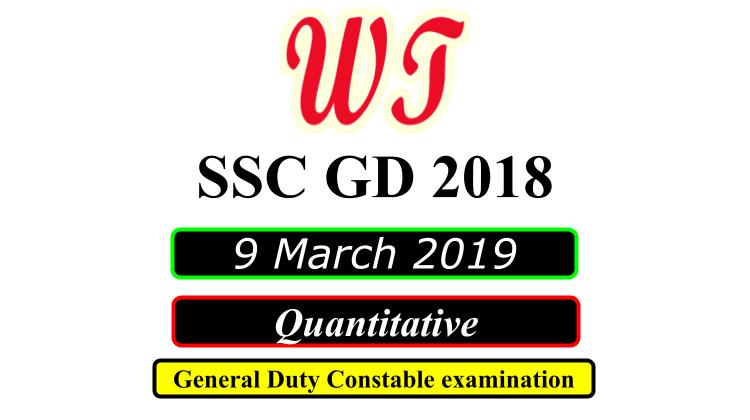Type Here to Get Search Results !

Download SSC (Staff Selection Commission) GD (General Duty), 11 March 2019 ( Math )Quantitative Questions PDF With Answers.

Table of Content

Table of Content (toc)SSC GD 9 March 2019 Quantitative Questions PDF Download Free

### SSC GD 9 March 2019 Shift 1 Math Questions

Q2:-A sold an article to B at a gain of 5% and B sold it to C at a gain of 10%.If C paid ₹ 462, what did it cost A?
A.    ₹400
B.    ₹500
C.    ₹480
D.    ₹320
Q3:-The radius of in-circle of a triangle is 4 cm and the perimeter of the triangle is 20 cm. What is the area of the triangle?
A.    40 sq. cm
B.    36 sq. cm
C.    80 sq. cm
D.    20 sq. cm
Q5:-What is the maximum number of students among whom 63 pens and 140 copies can be distributed in such a way that each student gets the same number of pens and same number of exercise books?
A.    5
B.    2
C.    7
D.    3
Q6:-A train passes a 60 metres long platform in 20 seconds and a man standing on the platform in 16 seconds. The speed of the train is:
A.    40 kmph
B.    50 kmph
C.    38 kmph
D.    54 kmph

### SSC GD 9 March 2019 Shift 2 Math Questions

Instructions
For the following questions answer them individually
Q1:-A shopkeeper organizes sale on Friday, offering a discount of 23% on all items. Still, he makes a profit of 10% only. By how much percentage CP was lower than MP?
A.    20%
B.    10%
C.    15%
D.    30%
Q2:-Train A took 30 minute to cover a distance of 50 km. If the speed of train B is 40% faster than train A, then the ratio of the respective speed of the both train is:
A.    5 : 3
B.    3 : 5
C.    7 : 5
D.    5 : 7
Q3:-The LCM of 779, 943 and 123 is:
A.    71668
B.    53751
C.    35834
D.    17917
Q5:-X is twice as good as workman as Y. Together, they finish the work in 18 days. In how many days can it be done by each separately?
A.    X = 21 days, Y = 42 days
B.    X = 9 days, Y = 18 days
C.    X = 19 days, Y = 38 days
D.    X = 27 days, Y = 54 days
Q7:-The sides of a triangle are in the ratio 6 : 4 : 3 and its perimeter is 104 cm. The length of the longest side (in cm)is:
A.    48
B.    44
C.    56
D.    120

### SSC GD 9 March 2019 Shift 3 Math Questions

Instructions
For the following questions answer them individually
Q1:-Study the chart and answer the question based, on the pie chart. The pie chart given below shows expenditures incurred by a family on various items and savings in a month. Savings of the family is ₹ 8,000 in a month.
What is the total expenditure of the family for the month?
A.    ₹45,000
B.    ₹48,000
C.    ₹50,000
D.    ₹40,000
Q2:-Nitin’s money becomes double in 4 years at compound interest. In how many years will it become sixteen times at compound Interest?
A.    20
B.    24
C.    16
D.    28
Q4:-What approximate value should come in place of the question mark (?) in the following questions?
A.    25
B.    35
C.    40
D.    10
Q6:-If 10 persons can do a job in 20 days. Then 20 persons with the twice efficiency can do the same job in:
A.    15
B.    10
C.    5
D.    20
Q7:-25% of a number is 300. The number is:
A.    1000
B.    800
C.    1200
D.    900

SSC GD 2018

SSC GD Previous Year Paper

SSC GD Previous Year English Questions

SSC GD Previous Year Reasoning Questions

SSC GD Previous Year Quantitative Questions